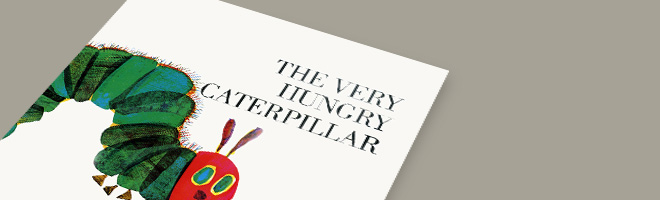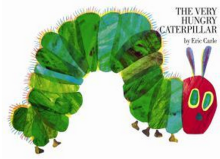• 09/08/13   |   Adjusted: 07/02/18   |   1 file# The Very Hungry Caterpillar

Author: Illustrative Mathematics

• Description
• Files

Mathematically:

• Develops students’ understanding of the relationship between counting on and addition (1.OA.C.5),
• Builds toward understanding of the place value system (1.NBT.B).
• Engages students in several Standards for Mathematical Practice (see Additional Thoughts).

In the classroom:

• Presents an application in an engaging setting
• Encourages students to talk about each other's thinking, in order to improve their mathematical understanding
• Allows for group or individual work

This task was designed to include specific features that support access for all students and align to best practice for English Language Learner (ELL) instruction. Go here to learn more about the research behind these supports. This lesson aligns to ELL best practice in the following ways:

• Provides opportunities for students to practice and refine their use of mathematical language.
• Allows for whole class, small group, and paired discussion for the purpose of practicing with mathematical concepts and language.
• Includes a mathematical routine that reflects best practices to supporting ELLs in accessing mathematical concepts.
• Provides opportunities to support students in connecting mathematical language with mathematical representations.

• Making the Shifts

How does this task exemplify the instructional Shifts required by CCSSM?

 Focus Belongs to the major work of first grade Coherence Builds on kindergarten work with addition Rigor Conceptual Understanding: secondary on this taskProcedural Skill and Fluency: not targeted in this taskApplication: primary in this task

Materials:The Very Hungry Caterpillar by Eric Carle

The students work individually or in pairs. Each student or pair needs:

• Three ten-frames for each student or pair of students (see PDF for black line master)
• 30 counters or unifix cubes per pair of students
• One small dry-erase board and dry-erase marker per pair of students

Actions:

The teacher reads the book to the class and asks, "How many things do you think the caterpillar ate in this story?" The students take a minute to share their estimate with a partner.

Next, the teacher reads The Very Hungry Caterpillar again. After each page, the teacher pauses so that the students can add counters or unifix cubes to the ten-frame to represent the number of things the caterpillar ate, and then write an equation on the dry-erase board connecting addition to the number of counters used. After each ten-frame is filled in the students move to the next one.

If the students are working in pairs, one student can add the counters/unifix cubes to the ten-frame while the other student writes the equation. By the end of the story, there should be a total of 25 food items eaten and one leaf eaten (the students can decide as a class whether to count the leaf as a food). There will be two ten-frames completed with five or six counters/unifix cubes on the third ten-frame.

If students come up with different, but correct, equations then discuss the different equations and ask students, "Can all of these be correct?"

• Illustrative Mathematics Commentary and Solution

Commentary:

The purpose of this is task is for students to solve word problems that call for addition of three whole numbers (1.OA.A.2), to relate counting on to addition (1.OA.C.5), and to understand that the two digits of a two-digit number represent amounts of tens and ones 1.NBT.B.2). This task supports developing conceptions of counting on and base-ten structure, and is thus appropriate early in the school year.

There is the possibility that students may write different, but correct, equations. If this happens, then the teacher should take the opportunity to ask students whether the different equations are correct and how they know. An appropriate classroom discussion can help support students' understanding of the equals sign (1.OA.D.7). While the standard only calls for sums within 20, in instructional situations it is appropriate to go beyond that. This limit is most salient for assessment developers.

Note that if this task is to support all these different standards, the teacher needs to be aware of the various connections and take the opportunity to draw them out as necessary.

Solution:

An example of what the students will be doing as the story is read:

After 1 apple and 2 pears are eaten, there will be 3 counters on the ten-frame. The equation will be $1+2=3$.

After 1 apple, 2 pears, and 3 plums are eaten, there will be 6 counters on the ten-frame. The equation could be either $3+3=6$ or $1+2+3=6$.

After 1 apple, 2 pears, 3 plums, and 4 strawberries are eaten, 4 more counters would be added to the ten-frame for a total of ten counters. The equation could be $6+4=10$, $3+3+4=10$, or $1+2+3+4=10$.

And so on!